#### 正文

calculator会做这样的事情：

``````如果你输入了：
2+2

3*3

……
``````

``````rails new calculator
cd calculator
``````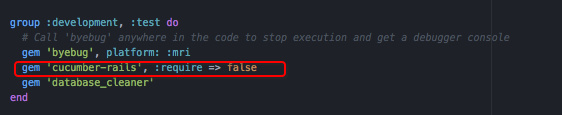database_cleaner这个gem是用来清理测试数据的，可加可不加，不过建议添加。

``````bundle install
rails g cucumber:install
``````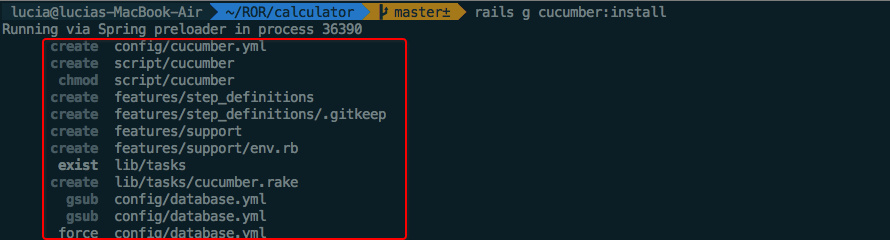``````rm -rf test
``````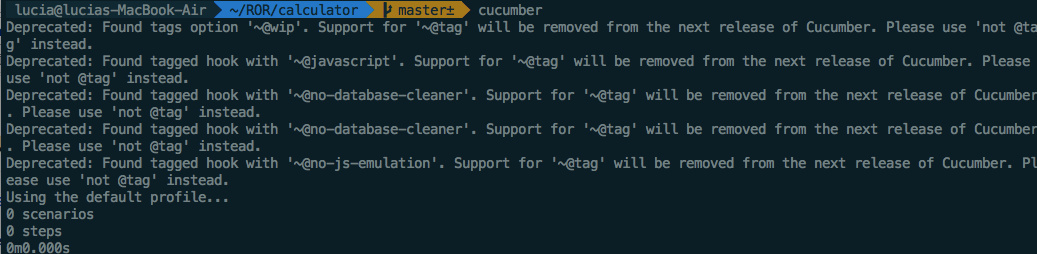``````touch features/adding.feature
``````

`adding.feature`文件的内容如下：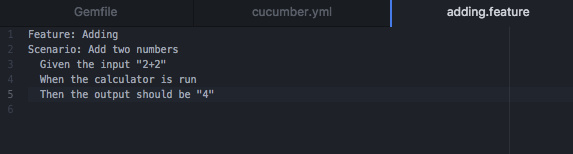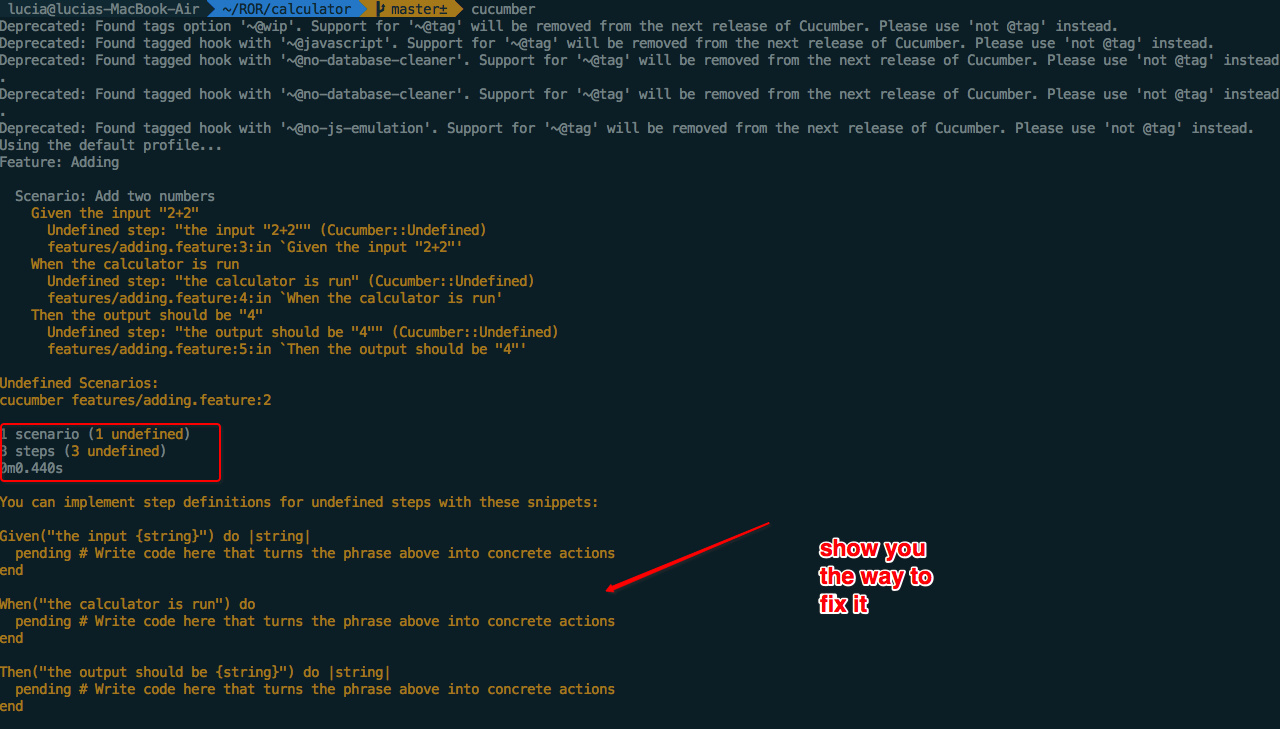【其实就是复制了它告诉我们的代码：P】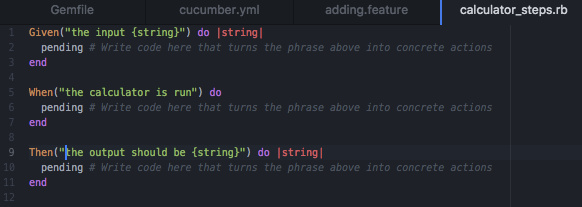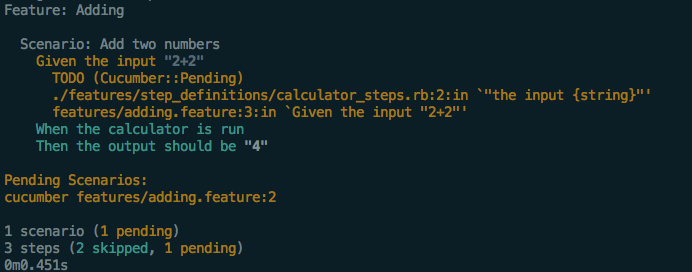``````Given("the input {string}") do |string|
@input = string
end

When("the calculator is run") do
pending # Write code here that turns the phrase above into concrete actions
end

Then("the output should be {string}") do |string|
pending # Write code here that turns the phrase above into concrete actions
end
``````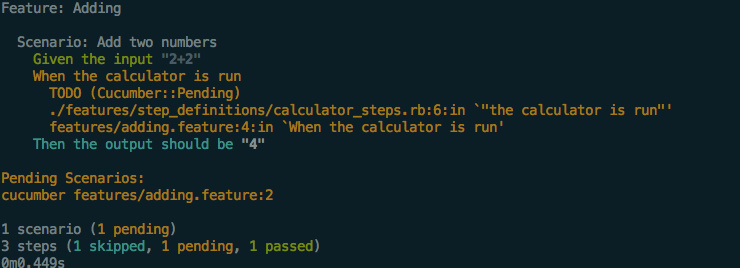``````Given("the input {string}") do |string|
@input = string
end

When("the calculator is run") do
@output = `ruby calc.rb #{@input}`
end

Then("the output should be {string}") do |string|
pending # Write code here that turns the phrase above into concrete actions
end
``````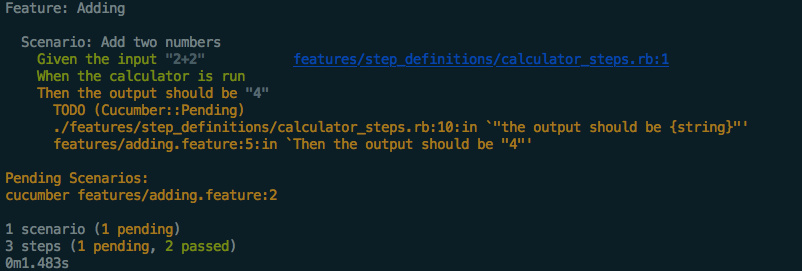``````Given("the input {string}") do |string|
@input = string
end

When("the calculator is run") do
@output = `ruby calc.rb #{@input}`
end

Then("the output should be {string}") do |string|
expect(@output).to eq string
end
``````

`calc.rb`中加入一行，使得output等于预期:

``````print eval (ARGV)
``````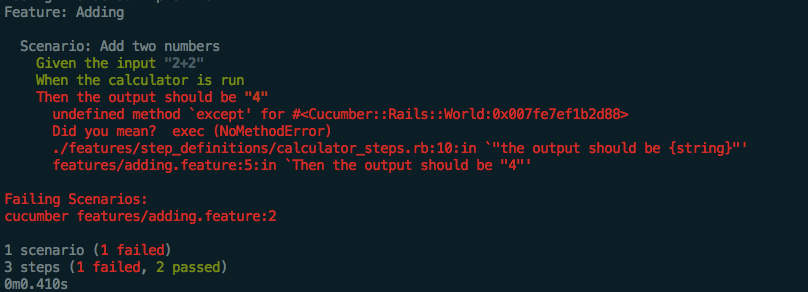``````gem 'rspec-rails'
``````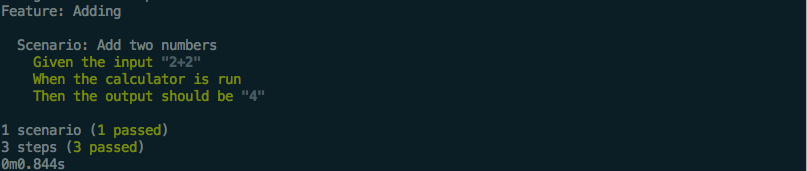cucumber的整个语法结构有个名字叫做Gherkin，例子中我们的`add.feature`就是遵守着Gherkin的语法。

Gherkin的大致结构类似这样：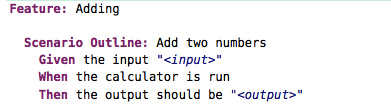#### The End

the cucumber book‘s forums

Cucumber：行为驱动开发指南